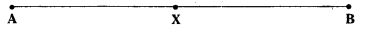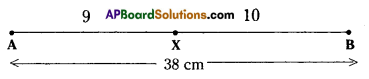# HSSlive: Plus One & Plus Two Notes & Solutions for Kerala State Board

## AP Board Class 7 Maths Chapter 6 Ratio Applications Ex 1 Textbook Solutions PDF: Download Andhra Pradesh Board STD 7th Maths Chapter 6 Ratio Applications Ex 1 Book AnswersAP Board Class 7 Maths Chapter 6 Ratio Applications Ex 1 Textbook Solutions PDF: Download Andhra Pradesh Board STD 7th Maths Chapter 6 Ratio Applications Ex 1 Book Answers

## Andhra Pradesh State Board Class 7th Maths Chapter 6 Ratio Applications Ex 1 Books Solutions

 Board AP Board Materials Textbook Solutions/Guide Format DOC/PDF Class 7th Subject Maths Chapters Maths Chapter 6 Ratio Applications Ex 1 Provider Hsslive

2. Click on the Andhra Pradesh Board Class 7th Maths Chapter 6 Ratio Applications Ex 1 Answers.
3. Look for your Andhra Pradesh Board STD 7th Maths Chapter 6 Ratio Applications Ex 1 Textbooks PDF.
4. Now download or read the Andhra Pradesh Board Class 7th Maths Chapter 6 Ratio Applications Ex 1 Textbook Solutions for PDF Free.

## AP Board Class 7th Maths Chapter 6 Ratio Applications Ex 1 Textbooks Solutions with Answer PDF Download

Find below the list of all AP Board Class 7th Maths Chapter 6 Ratio Applications Ex 1 Textbook Solutions for PDF’s for you to download and prepare for the upcoming exams:

Question 1.
What is the ratio of ₹ 100 and ₹ 10? Express your answer in the simplest form.
Solution:
Ratio of ₹ 100 and ₹ 10 = 100 : 10 = 10010=101 = 10 : 1
Simplest form = 10 : 1.

Question 2.
Sudha has ₹ 5. Money with Radha is 3 times the money with Sudha. How much money does Radha have?
(i) What is the ratio of Radha’s money and Sudha’s money?
(ii) What is the ratio of Sudha’s money and Radha’s money?
Solution:
Money with Sud ha = 5
∴ MoneywltbRadha = 3 times Sudha
= 3 × ₹5 = ₹ 15

i) Radha : Sudha 15 : 5
155=31
= 3 : 1

ii) Sudha:Radha = ₹ 5 : ₹ 15
= 5 : 15
= 515
= 1 : 3

Question 3.
Divide 96 chocolates between Raju and Ravi in the ratio 5 : 7
Solution:
Given ratio = 5 : 7
Sum of the terms of the ratio = 5 + 7 = 12
TotaL chocolates = 96
∴ Rajus share 512 x 96 = 40
Ravis share 712 x 96 = 56

Question 4.
The length of a line segment AB is 38 cm. A point X on it divides it in the ratio 9: 10. Find the lengths of the line segments AX and XB.Solution:Given ratio AX : XF = 9 : 10
Sum of the terms of the ratio = 9 + 10 = 19
Total length of the line segment = 38 cm
∴ AX⎯⎯⎯⎯⎯⎯⎯⎯⎯=919 × 38 = 18cm
XB⎯⎯⎯⎯⎯⎯⎯⎯=1019 × 38 = 20cm

Question 5.
A sum of ₹ 160,000 is divided in the ratio of 3 : 5. What is the smaller share’?
Solution:
Given that the sum is divided in the ratio = 3: 5
Sum divided = ₹ 1,60,000
Sum of the terms of the ratio 3 + 5 = 8
∴ Smaller share = 38 × 1,60.000 = ₹ 60,000

Question 6.
To make green paint, a painter mixes yellow paint and blue paint in the ratio of 3 :2. If he
used twelve liters of yellow paint, how much blue paint did he use ?
Solution:
Ratio of yellow paint and blue paint = 3: 2
Quantity of yellow paint = 3 parts = 12 litres
Quantity of blue paint = 2 parts = 23 × 12 = 8 litres

Question 7.
A rectangle measures 40 cm at its length and 20 cm at its width. Find the ratio of the length
to the width.
Solution:
Length of the rectangle = 40cm
Width of the rectangle = 20cm
Ratio of length and width = 40 cm : 20 cm
= 4020=21 = 2 : 1

Question 8.
The speed of a Garden-Snail is 50 meters per hour and that of the Cheetah is 120 kilometers per hour. Find the ratio of the speeds.
Solution:
Speed of the Garden Snell = 50 m/hour
= 501000 kmph
= 120 kmph
Speed of the Cheetah = 120 kmph
Ratio of their speeds = 120 : 120
= 2020 : 20 x 120
= 1 : 2400

Question 9.
Find (i) The ratio of boys and girls in your class.
(ii) The ratio of number of doors and number of windows of your classroom.
(ii) The ratio of number of text books and number of note books with you

## Andhra Pradesh Board Class 7th Maths Chapter 6 Ratio Applications Ex 1 Textbooks for Exam Preparations

Andhra Pradesh Board Class 7th Maths Chapter 6 Ratio Applications Ex 1 Textbook Solutions can be of great help in your Andhra Pradesh Board Class 7th Maths Chapter 6 Ratio Applications Ex 1 exam preparation. The AP Board STD 7th Maths Chapter 6 Ratio Applications Ex 1 Textbooks study material, used with the English medium textbooks, can help you complete the entire Class 7th Maths Chapter 6 Ratio Applications Ex 1 Books State Board syllabus with maximum efficiency.

## FAQs Regarding Andhra Pradesh Board Class 7th Maths Chapter 6 Ratio Applications Ex 1 Textbook Solutions

#### Can we get a Andhra Pradesh State Board Book PDF for all Classes?

Yes you can get Andhra Pradesh Board Text Book PDF for all classes using the links provided in the above article.

## Important Terms

Andhra Pradesh Board Class 7th Maths Chapter 6 Ratio Applications Ex 1, AP Board Class 7th Maths Chapter 6 Ratio Applications Ex 1 Textbooks, Andhra Pradesh State Board Class 7th Maths Chapter 6 Ratio Applications Ex 1, Andhra Pradesh State Board Class 7th Maths Chapter 6 Ratio Applications Ex 1 Textbook solutions, AP Board Class 7th Maths Chapter 6 Ratio Applications Ex 1 Textbooks Solutions, Andhra Pradesh Board STD 7th Maths Chapter 6 Ratio Applications Ex 1, AP Board STD 7th Maths Chapter 6 Ratio Applications Ex 1 Textbooks, Andhra Pradesh State Board STD 7th Maths Chapter 6 Ratio Applications Ex 1, Andhra Pradesh State Board STD 7th Maths Chapter 6 Ratio Applications Ex 1 Textbook solutions, AP Board STD 7th Maths Chapter 6 Ratio Applications Ex 1 Textbooks Solutions,
Share: